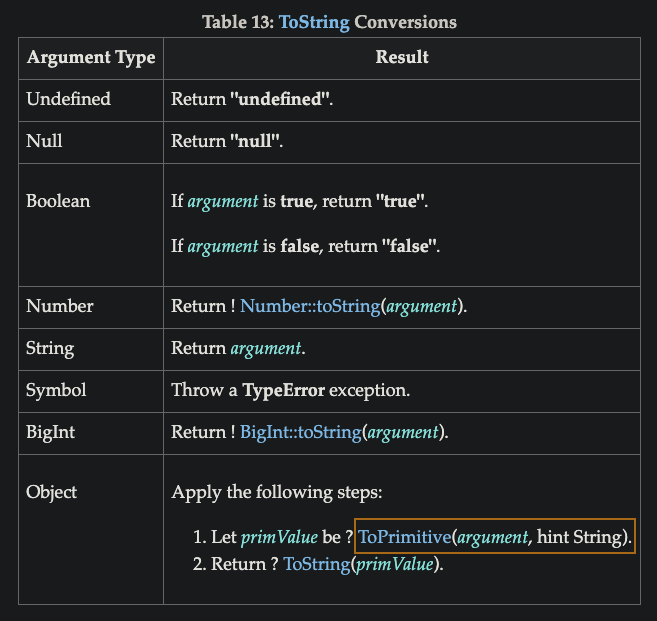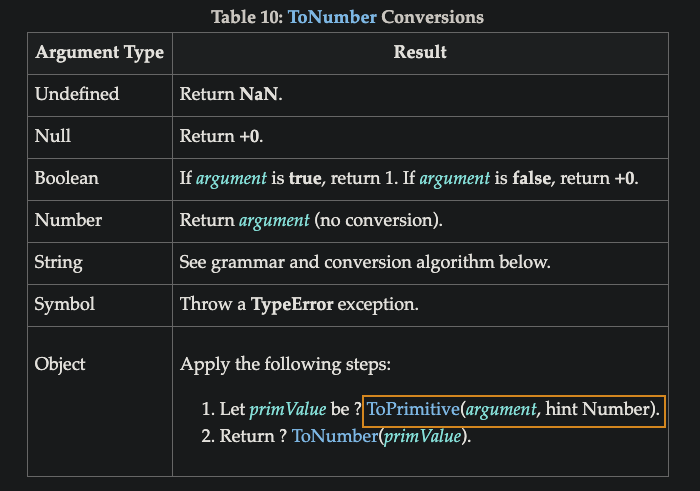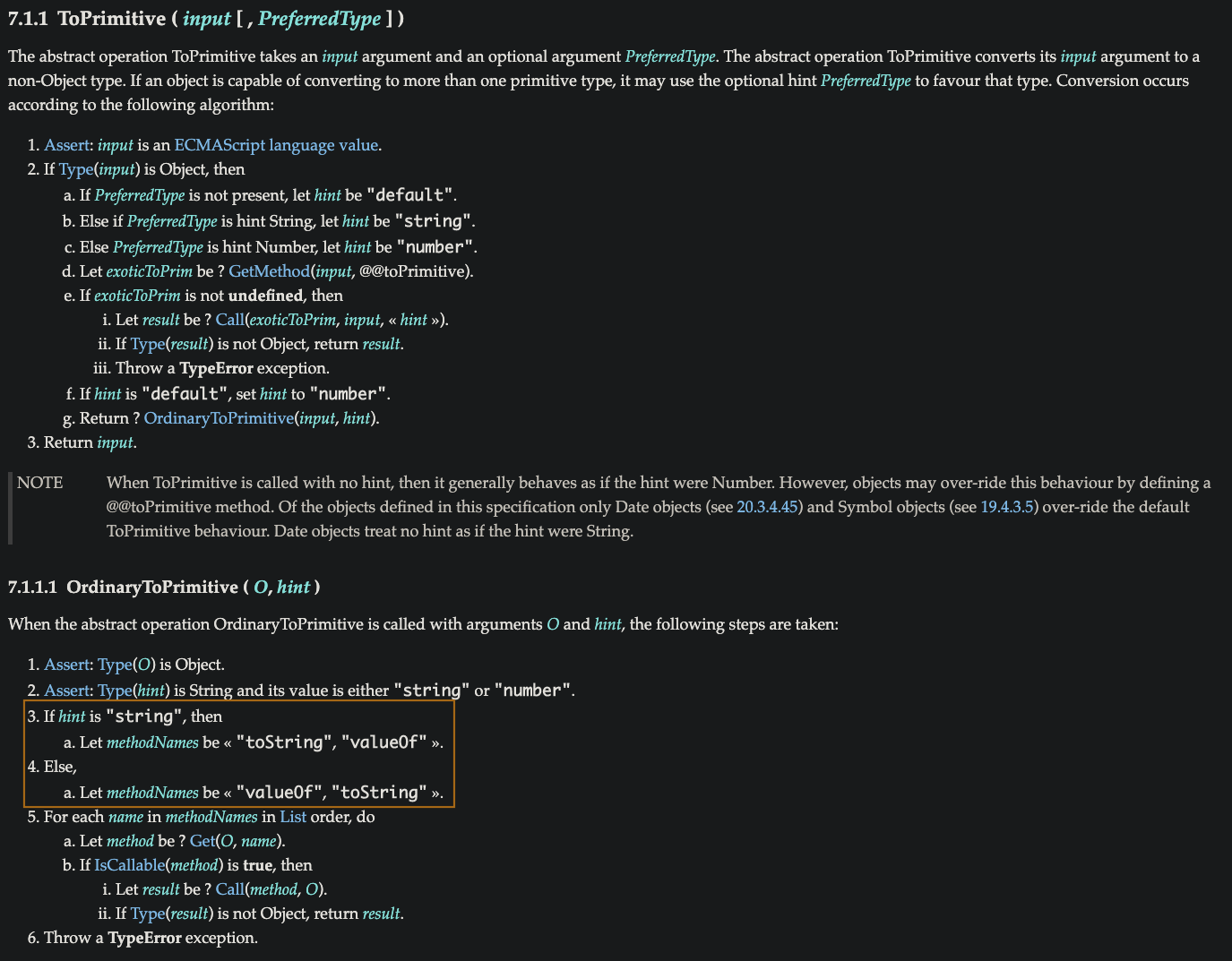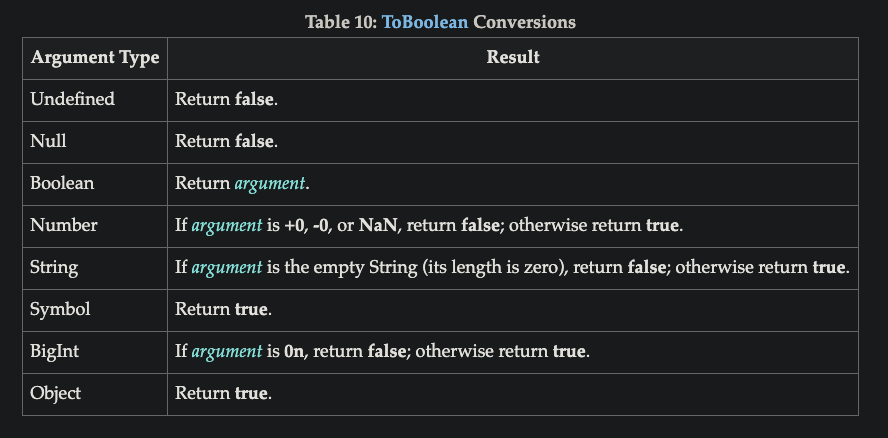#  強制轉型 - parseInt、Number、ToPrimitive、Boolean

keywords:`parseInt`,`Number`,`ToPrimitive`,`Boolean`

### 明確地：剖析數值字串

``````    let a = '99';

let b = '99px';

Number(a); // 99

parseInt(a); // 99

Number(b); // NaN

parseInt(b); // 99
``````
• parseInt：一個字串剖析出數值能容忍非數值字元，從左到右進行剖析，遇到字元時單純就停下來，如果不在意可能會有非數值字元可以用 parse 剖析為 number
• Number：強制轉型如果有字元，轉換會失敗，產生 NaN 值，如果只能接受數字認為 '99PX' 不該被視為數字，就使用強制轉型 coerce

parseInt(…) 只能作用於 string 值，傳入非 string 值則是毫無意義的，如果串入一個非字串，會先自動隱含強制轉型為一個 string ( 使用 ToString ) ，所以永遠都不要把非字串值傳入 parseInt(..)

``````    parseInt(true); // NaN

parseInt(function(){}); // NaN

parseInt([1,2,3]); // 1

[1,2,3] 會先被 toString() 隱含強制轉成 '1,2,3' 字串，然後進行 parseInt('1,2,3') 通過 1 後遇到 , 所以就會停下來回傳 1

parseInt([,2,3]); // NaN
``````

parseInt(..) 有一個雙生的 parseFloat(..)，會從一個字串剖析出一個浮點數字

``````    parseInt('1.254'); // 1

parseFloat('1.254'); // 1.254
``````
• ES5 之前 parseInt(..) 有一個陷阱，也可以說是 JS 臭蟲的來源，如果沒有傳入第二個引數指出要用來解讀數值 string 內容的基數 ( radix )，parseInt(..) 會檢視開頭的那幾個字元猜測
• 開頭 "0x" 或 "0X" 會猜測要解讀為一個十六進位 ( hexadecimal base-16 ) 的 number
• 開頭 "0" 會猜測為要解讀為一個八進位 ( octal base-8 ) 的 number
• 🚩所以使用 parseInt(..) 有幾點要特別注意
1.永遠都要傳入 10 ( ES5 中 假設基數為 10 ) 做為第二個引數
2.永遠傳入字串，不然就會被隱含的強制轉型為 string

### 剖析非字串

``````    parseInt( 1/0 ,19); // 18

首先 1 / 0 會是 Infinity 但因為是非字串，故會先轉成字串 'Infinity'

parseInt("Infinity",19) 而 'I' 在 base-19 裡的值就是 18

第二個 n 不在有效數值字元的集合，因此剖析就會禮貌地停止，就像 "99px" 中遇到 "p" 時那樣

這裡犯下最明顯的過失就是傳入一個非字串給 parseInt(..)，不過 JS 還是很有禮貌的將傳入的東西強制轉型為 string 並進行剖析
``````

``````    parseInt(new String('99')); // 99

如果是物件，會先執行物件包裹器解封 ( unboxed ) ToPrimitive()，new String('99') 就會變成 '99'
``````• ToString：object 要轉型至 string 會呼叫 ToPrimitive hint 為 String
• ToNumber：object 要轉型至 number 會呼叫 ToPrimitive hint 為 Number
• ToPrimitive 7.1.1.1 顯示：
hint String 優先順序就是 toString() => valueOf()
hint Number 優先順序就是 valueOf() => toString()

``````  let a = {

num: 38,

toString: function(){ return String(this.num * 2) },

valueOf: function() { return Number(this.num / 2) }

}

parseInt(a); // 76

Number(a); // 19

∵ parseInt() 會先強制轉型為一個 string （ 使用 ToString ）

Number() 則是強制轉型為一個 number（ 使用 ToNumber ）

ㄎ 還蠻有趣的 🤣
``````

### 明確地 any > Boolean

• 就跟 String()、Number() 一樣，現在是任何非 Boolean 值強制轉型為一個 boolean強制轉型 - ToBoolean、Falsy、Truthy 就有整理五項 8 個 falsy 值

• 如同單元 ( unary ) 的 + 會把一個值強制轉型為一個 number，單元的 ! 否定運算子 ( negate operator ) 也會明確地將一個值強行轉型為 boolean ，但問題在於它也會反轉 ( flip ) 該值，把它從 truthy 變為 falsy ，或者相反

``````  let a = 1;

!a; // false

let b = 0;

!b; // true
``````
• 因此 JS 開發人員明確將值強制轉型為 boolean 最常見的方式是使用 !! 雙否定運算子，因為第二個 ! 會把真假值 ( parity ) 反轉回原來的狀態

``````  let a = "0";

let b = [];

let c = {};

let d = "";

let e = 0;

let f = null;

let g;

!!a; // true

!!b; // true

!!c; // true

!!d; // false

!!e; // false

!!f; // false

!!g; // false
``````
• ? : 這個三元運算子 ( ternary operator ) 有一個難以察覺的隱含強制轉型：

`````` let a = 98;

a? true : false; // true
``````

a 運算式必須先被強制轉型為 boolean，才能進行真假值的測試。
這種慣用法叫做明確地隱含 ( explicitly implicit )，應該要在程式裡完全避免這種慣用法，有時候會偽裝成別的東西

• 就明確強制轉型而言， Boolean ( a ) 和 !!a 都是好得多的選擇
#parseInt #number #Toprimitive #Boolean